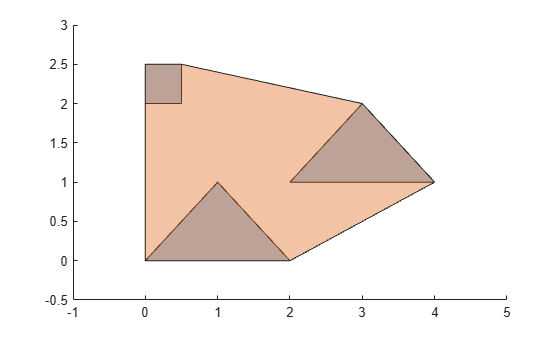convhull

Convex hull of polyshape

Description

example

polyout = convhull(polyin) returns the convex hull of a polyshape object. polyout is a polyshape object or an array of polyshape objects the same size as polyin.

Examples

collapse all

Compute the convex hull of a polygon containing three solid regions.

x1 = [0 1 2];
y1 = [0 1 0];
x2 = [2 3 4];
y2 = [1 2 1];
x3 = [0 0.5 0.5 0];
y3 = [2 2 2.5 2.5];
polyin = polyshape({x1,x2,x3},{y1,y2,y3});
plot(polyin)
hold on
polyout = convhull(polyin);
plot(polyout)Input Arguments

collapse all

Input polyshape, specified as a scalar, vector, matrix, or multidimensional array.

Data Types: polyshape